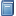Buchempfehlung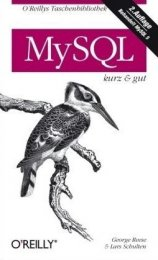MySQL kurz & gut
Das preiswerte Taschen- buch stellt MySQL-rele- vante Inhalte systematisch und knapp dar, sodass es sich optimal zum Nach- schlagen beim Pro- grammieren eignet. [Mehr Infos...]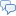FreeBASIC-Chat
Es sind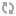Benutzer im FreeBASIC-Chat online.
(Stand:  )

# fb:porticula NoPaste

## GuiWindow.bi

 Uploader: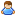OneCypher Datum/Zeit: 13.10.2009 11:05:53
Hinweis: Dieser Quelltext ist Bestandteil des Projekts GuiPtr, zu dem es auf FreeBASIC-Portal.de eine Projektseite gibt.

```#include once "GuiPtr.bi" #include once "ScrollBar.bi" type NullWindow     Object as GuiObject ptr     BackColor as uinteger = RGB(58,110,165)     BorderColor as ubyte     BorderStyle as ubyte     VScrollbar as Scrollbar ptr     HScrollbar as Scrollbar ptr     dX as integer     dY as integer     declare sub StartThread()     declare sub QuitThread()     declare sub SleepThread(t as integer = 1)     declare sub ThreadLock()     declare sub ThreadUnlock()     Declare function AddVScrollbar() as Scrollbar ptr     Declare function AddHScrollbar() as Scrollbar ptr     declare constructor(Title as string = "")     declare constructor overload(l as integer, t as integer, w as integer, h as integer) end type Sub MoveElements(GO as any ptr, e as EventParameter)     dim w as GuiObjectControl ptr = GO     dim o as GuiObject ptr = w->GuiObjectPTR     dim c as GuiObject ptr     ForEach(c) in(o->ChildObjects)         if c->FixedPosition = 0 then             c->left += e.mdx             c->top += e.mdy         end if     NextOne end sub Sub CalcInnerSizes(GO as any ptr, e as EventParameter)     dim nw as NullWindow ptr = go     Dim Child as GuiObject ptr     'dim dX as integer     'dim dY as integer     dim dXMin as integer     Dim dYMin as integer     dim dXMax as integer     Dim dYMax as integer     for i as integer = 1 to nw->Object->ChildObjects.Count         Child = nw->Object->ChildObjects.Item(i)         if Child->Enabled = 1 then             if nw->HScrollbar <> 0 then                 if Child->left < dXMin then dXMin = Child->left                 if Child->left + Child->width > nw->Object->Width then dXMax = Child->left + Child->width - nw->Object->Width             end if             if nw->VScrollbar <> 0 then                 if Child->top < dYMin then dYMin = Child->top                 if Child->top + Child->Height > nw->Object->Height then dYMax = Child->top + Child->Height - nw->Object->Height             end if         end if     next     if nw->HScrollBar <> 0 then         nw->dX = dXMax - dXMin  'Entspricht 100         nw->HScrollBar->MinValue = dXMin         nw->HScrollBar->MaxValue = dXMax         nw->HScrollBar->Value = 0     end if     if nw->VScrollBar <> 0 then         nw->dY = dYMax - dYMin  'Entspricht 100         nw->VScrollBar->MinValue = dYMin         nw->VScrollBar->MaxValue = dYMax         nw->VScrollBar->Value = 0     end if     'if nw->VScrollbar <> 0 then     '    Dump "MinY= " &nw->VScrollBar->MinValue     '    Dump "MaxY= " &nw->VScrollBar->MaxValue     '    Dump "   Y= " &nw->VScrollBar->Value     'else     '    Dump "NoVScroll"     'end if end sub Sub DragVScrollbar(GO as any ptr, e as EventParameter)     dim vsb as Scrollbar ptr = go     dim nw as NullWindow ptr = vsb->Object->Parent->MyObject     Dim TmpE as EventParameter     TmpE.mdy = -1* vsb->Value     MoveElements nw, TmpE     CalcInnerSizes nw, e     nw->Object->root->Redraw end sub function nullWindow.AddVScrollbar() as Scrollbar ptr     if VScrollbar = 0 then         dim vsb as Scrollbar ptr = New Scrollbar(Object->width - 18,0,18,Object->Height - Object->ClientTop)         vsb->Object->AlwaysOnTop = 1         vsb->Object->FixedPosition = 1         vsb->Object->PublicEvents->OnMouseDrag = @ DragVScrollbar         vsb->Object->PublicEvents->SingleClick = @ DragVScrollbar         VScrollbar = Object->Add(vsb)     end if     return VScrollbar end function Sub DragHScrollbar(GO as any ptr, e as EventParameter)     dim hsb as Scrollbar ptr = go     dim nw as NullWindow ptr = hsb->Object->Parent->MyObject     Dim TmpE as EventParameter     TmpE.mdx = -1* hsb->Value     MoveElements nw, TmpE     CalcInnerSizes nw, e     nw->Object->root->Redraw end sub function nullWindow.AddHScrollbar() as Scrollbar ptr     If HScrollBar = 0 then         dim hsb as Scrollbar ptr = New Scrollbar(0,Object->Height -18, Object->Width,18)         hsb->Object->Ignoreclient = 1         hsb->Object->AlwaysOnTop = 1         hsb->Object->FixedPosition = 1         hsb->Object->PublicEvents->OnMouseDrag = @ DragHScrollbar         hsb->Object->PublicEvents->SingleClick = @ DragHScrollbar         HScrollbar = Object->Add(hsb)     end if     Return HScrollbar end function type GuiWindow     Object as GuiObject ptr     title as string     Icon as any ptr     SafeLeft as integer     SafeTop as integer     SafeWidth as integer     SafeHeight as integer     maximized as integer     ForeColor as uinteger = RGB(217,229,242)     BackColor as uinteger = RGB(212,208,200)     BorderStyle as integer     declare sub StartThread()     declare sub QuitThread()     declare sub SleepThread(t as integer=1)     declare sub ThreadLock()     declare sub ThreadUnlock()     declare constructor(left as integer, top as integer, w as integer, h as integer, GWindowtitle as string)     'testvariablen     mx as integer     my as integer end type Sub GuiWinResize(GO as any ptr, e as EventParameter)     dim w as GuiObjectControl ptr = GO     dim o as GuiObject ptr = w->GuiObjectPTR     dim oldbuffer as any ptr     o->width  += e.mdx     o->height += e.mdy     oldbuffer = o->Buffer     Imagedestroy o->Buffer     o->Buffer = imageCreate(o->width+1, o->height +1,RGB(0,0,0))     o->root->ChangeBuffer oldbuffer, o->Buffer     o->PrivateEvents->EmitDraw(Go) end sub Sub ObjectResize(GO as any ptr, e as EventParameter)     dim w as GuiObjectControl ptr = GO     dim o as GuiObject ptr = w->GuiObjectPTR     dim oldbuffer as any ptr     o->width  += e.mdx     o->height += e.mdy     oldbuffer = o->Buffer     Imagedestroy o->Buffer     o->Buffer = imageCreate(o->width+1, o->height +1,RGB(0,0,0))     o->root->ChangeBuffer oldbuffer, o->Buffer end sub Sub GuiWinMove(GO as any ptr, e as EventParameter)     dim w as GuiObjectControl ptr = GO     dim o as GuiObject ptr = w->GuiObjectPTR         o->left += e.mdx         o->top += e.mdy end sub Sub MaximizeWindow(GO as any ptr, e as EventParameter)     dim w as GuiWindow ptr = GO     dim as integer sx, sy     screencontrol 4, sx, sy     dim oldbuffer as any ptr     if e.my < 22 then         if w->maximized then             w->maximized = 1<>1             w->object->left = w->SafeLeft             w->object->Top = w->SafeTop             w->object->Width = w->SafeWidth             w->object->Height = w->SafeHeight             oldbuffer = w->object->buffer             imagedestroy w->object->buffer             w->object->Buffer = imagecreate(w->object->Width+1,w->object->Height+1)         else             w->maximized = 1<>0             w->SafeLeft = w->object->left             w->SafeTop = w->object->Top             w->SafeWidth = w->object->Width             w->SafeHeight = w->object->Height             w->object->left = 0             if w->object->parent = 0 then                 w->object->Top = 0                 w->object->Width = sx                 w->object->Height = sy             else                 w->object->Top = w->object->parent->ClientTop                 w->object->Width = w->object->parent->width                 w->object->height = w->object->parent->height - w->object->parent->ClientTop             end if             oldbuffer = w->object->buffer             imagedestroy w->object->buffer             w->object->Buffer = imagecreate(sx+1,sy+1)         end if         w->object->ChangeBuffer oldbuffer, w->object->buffer         w->object->root->redraw     end if end sub Sub NullWinBehavior(GO as any ptr, e as EventParameter)     if e.mb = 3 then         MoveElements GO, e     end if end sub sub GuiWinBehavior(GO as any ptr, e as EventParameter)     dim w as GuiWindow ptr = GO     if w->Maximized = 0 then         if e.mb = 1 then GuiWinMove GO, e         if e.mb = 2 then GuiWinResize GO, e         if e.mb = 3 then MoveElements GO, e     end if end sub Sub ReDrawGuiWin(GWindowPTR as any ptr)     dim w as GuiWindow ptr = GWindowPTR     with *w         with *w->Object             line .buffer, (0,0)-(.width,.height), w->BackColor, BF             'line .buffer, (0,0)-(.width, 22 ), RGB(166,202,240), BF             line .buffer, (0,0)-(.width, 22 ), w->ForeColor, BF             if w->BorderStyle = 0 then line .buffer, (0,0)-(.width,.height), RGB(0,0,0), B             if w->BorderStyle = 1 then                 line .buffer, (0, 0)-(0 + .width, 0 + .height),RGB(64,64,64),B                 line .buffer, (0, 0)-(0, 0 + .height),RGB(255,255,255)                 line .buffer, (0, 0)-(0 + .width, 0),RGB(255,255,255)             end if             'line .buffer, (0,23)-(.width,23), RGB(255,255,255)             'line .buffer, (0,23)-(0, .height), RGB(255,255,255)             if w->Icon <> 0 then                 draw string .buffer, (23, 5), w->title,RGB(0,0,0)                 line .buffer, (4,4)-(18, 18),RGB(255,255,255),BF             else                 draw string .buffer, (6, 5), w->title,RGB(0,0,0)             end if         end with     end with     'line w->Object->Buffer,(0,0)-(w->mx,w->my),RGB(255,0,0) end sub Sub RedrawNullWindow(GO as any ptr)     dim nw as NullWindow ptr = GO     with *nw->Object         line .buffer,(0,0)-(.width,.height),nw->BackColor,BF         'Dump "Ist:  " & str(nw->BackColor)         'dump "Soll: " & str(RGB(255,255,255))         select case nw->BorderStyle         Case 0         case 1             line .buffer, (0, 0)-(0 + .width, 0 + .height),nw->BorderColor,B         case 2             line .buffer, (0, 0)-(0 + .width, 0 + .height),RGB(64,64,64),B             line .buffer, (0, 0)-(0, 0 + .height),RGB(255,255,255)             line .buffer, (0, 0)-(0 + .width, 0),RGB(255,255,255)         end select     end with end sub 'Threadhandles: Sub WindowThread(GO as any ptr)     dim W as NullWindow ptr = GO     w->Object->Exec end sub Sub GuiWindow.QuitThread()     MutexLock Object->ThreadMutex     Object->ThreadCancel = 1     MutexUnLock Object->ThreadMutex     ThreadWait Object->ThreadID end sub sub GuiWindow.ThreadLock()     mutexlock Object->ThreadMutex end sub sub GuiWindow.ThreadUnlock()     mutexunlock Object->ThreadMutex end sub sub GuiWindow.SleepThread(t as integer = 1)     MutexLock Object->ThreadMutex     Sleep t     MutexUnLock Object->ThreadMutex end sub Sub GuiWindow.StartThread()     Object->ThreadID = ThreadCreate(Cast(Any Ptr,@WindowThread), @This)     Object->ThreadMutex = MutexCreate end sub Sub NullWindow.QuitThread()     MutexLock Object->ThreadMutex     Object->ThreadCancel = 1     MutexUnLock Object->ThreadMutex     ThreadWait Object->ThreadID end sub sub NullWindow.ThreadLock()     mutexlock Object->ThreadMutex end sub sub NullWindow.ThreadUnlock()     mutexunlock Object->ThreadMutex end sub sub NullWindow.SleepThread(t as integer = 1)     MutexLock Object->ThreadMutex     Sleep t     MutexUnLock Object->ThreadMutex end sub Sub NullWindow.StartThread()     Object->ThreadID = ThreadCreate(Cast(Any Ptr,@WindowThread), @This)     Object->ThreadMutex = MutexCreate end sub constructor NullWindow(Title as string = "")     dim as integer sx, sy     screencontrol 4, sx, sy     If Title < "" then WindowTitle Title     Object = new GuiObject(@This)     with *Object         .Name = "NullWindow"         .ClassName = "NullWindow"         .left = 0         .top = 0         .width = sx         .height = sy         .PrivateEvents = new Events         .PrivateEvents->OnDraw = @RedrawNullWindow         .PrivateEvents->OnMouseDrag = @NullWinBehavior         .buffer = imagecreate(sx+1,sy+1,RGB(0,0,128))         .DrawPriority = 10     end with end constructor constructor NullWindow(l as integer, t as integer, w as integer, h as integer)     Object = new GuiObject(@This)     with *Object         .Name = "NullWindow"         .ClassName = "NullWindow"         .left = l         .top = t         .width = w         .height = h         .PrivateEvents = new Events         .PrivateEvents->OnDraw = @RedrawNullWindow         .PrivateEvents->OnMouseDrag = @NullWinBehavior         .buffer = imagecreate(w+1,h+1,RGB(0,0,128))     end with     'Controlelements end constructor constructor GuiWindow(left as integer, top as integer, w as integer, h as integer, GWindowtitle as string)     Object = new GuiObject(@This)     with *Object         .Name = "GuiWindow"         .ClassName = "GuiWindow"         .left = left         .top = top         .width = w         .height = h         .ClientTop = 23         .buffer = imagecreate(w+1,h+1)         .PrivateEvents = new Events         .DrawPriority = 2         with *.PrivateEvents             .OnDraw = @ReDrawGuiWin             .OnMouseDrag = @GuiWinBehavior             .DoubleClick = @MaximizeWindow         end with     end with     'Controlelements     Title = GWindowTitle end constructor```